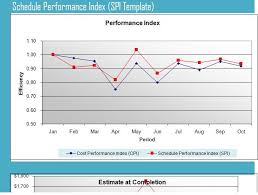## How to Calculate and Solve for Performance Index | Material SelectionThe image above represents performance index.

To compute for performance index, two essential parameters are needed and these parameters are Shear Stress at Fracture (τf) and Density (ρ).

The formula for calculating the performance index:

P = τf2/3 / ρ

Where:

P = Performance Index
τf = Shear Stress at Fracture
ρ = Density

Let’s solve an example;/
Find the performance index when the shear stress at fracture is 32 and the density is 10.

This implies that;

τf = Shear Stress at Fracture = 32
ρ = Density = 10

P = τf2/3 / ρ
P = 322/3/10
P = 10.079 / 10
P = 1.0079

Therefore, the performance index is 1.0079.